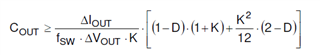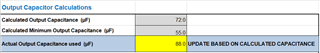If you have a related question, please click the "Ask a related question" button in the top right corner. The newly created question will be automatically linked to this question.

# LMR33630-Q1: Output Capacitance equation

Part Number: LMR33630-Q1
Other Parts Discussed in Thread: LMR33630

Hi all

I found the LMR336 calculator from TI, I have checked from datasheet, but there is only one equation for Cout :And when I try to do manual calculation, the result is different with calculator one.

I would like to know what formula is used for Calculated Output Capacitance and Calculated Minimum Output Capacitance in this calculator:Attached are the datasheet and calculator that i found

Thank you :)

• Hi,

The equation used in the calculation tool is the same as in the datasheet. Put ΔIout=2A, Fsw=400k, ΔVout=250mV, k=0.3, D=5/12, you can get Cout=52uF. Assuming a 20% tolerance and a 10% bias de-rating, you can get Cout=52/0.8/0.9=72uF.

Regards,

Hongjia

• Hi Hongjia,

I have check the equation and its work for both Calculated Output Capacitance and Calculated Minimum Output Capacitance.

But i would like to know for this calculation :Cout=52/0.8/0.9=72uF. You said we assuming a 20% tolerance and a 10% bias de-rating to get the cout, so where is 0.8 and 0.9 come from ?

Thank you

• Hi,

1-20%=0.8 and 1-10%=0.9.

Regards,

Hongjia